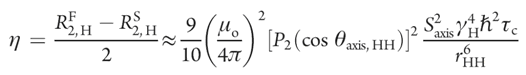# Methyl sidechain S2 measurement

Notes for measurement of side-chain order in methyl-protonated proteins

### 1. In order to extract the intra-methyl 1H-1H dipolar cross-correlated relaxation rate η, CH3_forbiddenDQ_allowed_800_lek.c pulse sequence (Tugarinov et al. JACS 2007, 129, 1743-1750.) was used to measure a series of pairs of hmqc-type exps.

2. Use nmrpipe to process all spectra with the same macro parameters and use nmrview to extract all peaks intensity values.

3. Load the data in excel, and get the Ia/Ib ratios, and draw the profile curves.

4. Use Igor (or K-graph or any) program to do the non-linear curve fitting to the equation (13) in the above paper, and extract the η values for every peaks.

5. Based on the following equation (Sun et al. J.Phys.Chem.B 2011, 115, 14878-14884.), calculate the order parameter S2axis.Where as:

RF2,H : fast transverse relaxation rate,

RS2,H : slow transverse relaxation rate,

μ0 : 1.2566E-6 T*m/A, ideal vacuum apedimity constant, (not ε0)

γH: 2.675E8 s-1*T-1, proton gyromagnetic ratio,

rHH: 1.813 E-10 m, the distance between pairs of methyl protons,

h: 6.626E-34 J*s, Planck constant,

τc: xxE-9 s, the global molecular tumbling time, (if you have not measured your protein’s τc, use the following website to calculate the rough value, http://nickanthis.com/tools/tau),

η: s-1, 1H-1H dipolar cross-correlated relaxation rate,

P2(cosθaxis,HH ) = P2(x) = ½(3x2-1)=- ½, where θaxis,HH is the angle between the methyl 3-fold axis and a vector connecting a pair of methyl 1H nuclei,

S2axis = 4*(10/9)*(4π /μ0)2* r6HH*η/(γ4H *(h/2π)2*τc)

For apoCupA, MW=11.2 kDa, τc = 7 ns, η = 16.862,

S2axis = (40*16*4*π4*1.8136E-60*16.862)/(9*1.25562*E-12*2.6754*E32*6.6262*E-68*7E-9)

= (40*16*4*π4 *1.8136*16.862*E-60)/( 9*1.25562*2.6754*6.6262*7*E-57)

= (43166.72*π4 *1.8136*E-3)/(63*1.25562*2.6754*6.6262)

= (43.16672*97.4091*35.513)/(63*1.5765*51.203*43.9039)

= 149326.17556/(63*1.5765*51.203*43.9039)

= 0.669 —peak4 for M91

Hongwei updated on 9/30/2014
created on Jan. 03, 2014